# 11: Hypothesis Testing

using computer simulation. Based on examples from the infer package. Code for Quiz 13.

Load the R package we will use.

``````library(tidyverse)
library(infer)
library(skimr)
``````
• Replace all the instances of ???. These are answers on your moodle quiz.

• Run all the individual code chunks to make sure the answers in this file correspond with your quiz answers

• After you check all your code chunks run then you can knit it. It won’t knit until the ??? are replaced

• Save a plot to be your preview plot

# Question: t-test

• The data this quiz is a subset of HR
• Look at the variable definitions
• Note that the variables evaluation and salary have been recoded to be represented as words instead of numbers
• Set random seed generator to 123

set.seed(123)

SEE QUIZ is the name of your data subset

• Read it into and assign to hr

• Note: col_types = “fddfff” defines the column types factor-double-double-factor-factor-factor
``````hr  <- read_csv("https://estanny.com/static/week13/data/hr_2_tidy.csv",
col_types = "fddfff")
``````
``````skim(hr)
``````
 Name hr Number of rows 500 Number of columns 6 _______________________ Column type frequency: factor 4 numeric 2 ________________________ Group variables None

Variable type: factor

skim_variable n_missing complete_rate ordered n_unique top_counts
gender 0 1 FALSE 2 mal: 256, fem: 244
evaluation 0 1 FALSE 4 bad: 154, fai: 142, goo: 108, ver: 96
salary 0 1 FALSE 6 lev: 95, lev: 94, lev: 87, lev: 85
status 0 1 FALSE 3 fir: 194, pro: 179, ok: 127

Variable type: numeric

skim_variable n_missing complete_rate mean sd p0 p25 p50 p75 p100 hist
age 0 1 39.86 11.55 20.3 29.60 40.2 50.1 59.9 ▇▇▇▇▇
hours 0 1 49.39 13.15 35.0 37.48 45.6 58.9 79.9 ▇▃▂▂▂

# Q: Is the mean number of hours worked per week 48?

specify that hours is the variable of interest

``````hr  %>%
specify(response = hours)
``````
``````Response: hours (numeric)
# A tibble: 500 x 1
hours
<dbl>
1  78.1
2  35.1
3  36.9
4  38.5
5  36.1
6  78.1
7  76
8  35.6
9  35.6
10  56.8
# … with 490 more rows``````

hypothesize that the average hours worked is 48

``````hr  %>%
specify(response = hours)  %>%
hypothesize(null = "point", mu = 48)
``````
``````Response: hours (numeric)
Null Hypothesis: point
# A tibble: 500 x 1
hours
<dbl>
1  78.1
2  35.1
3  36.9
4  38.5
5  36.1
6  78.1
7  76
8  35.6
9  35.6
10  56.8
# … with 490 more rows``````

generate 1000 replicates representing the null hypothesis

``````hr %>%
specify(response = hours)  %>%
hypothesize(null = "point", mu = 48)  %>%
generate(reps = 1000, type = "bootstrap")
``````
``````Response: hours (numeric)
Null Hypothesis: point
# A tibble: 500,000 x 2
# Groups:   replicate [1,000]
replicate hours
<int> <dbl>
1         1  33.9
2         1  78.5
3         1  35.8
4         1  34.2
5         1  52.9
6         1  36.9
7         1  72.7
8         1  33.6
9         1  72.7
10         1  50.2
# … with 499,990 more rows``````

The output has 500,000 rows

calculate the distribution of statistics from the generated data

• Assign the output null_t_distribution

• Display null_t_distribution

``````null_t_distribution  <- hr  %>%
specify(response = age)  %>%
hypothesize(null = "point", mu = 48)  %>%
generate(reps = 1000, type = "bootstrap")  %>%
calculate(stat = "t")

null_t_distribution
``````
``````# A tibble: 1,000 x 2
replicate     stat
*     <int>    <dbl>
1         1  0.754
2         2 -0.403
3         3  0.287
4         4  0.373
5         5  0.00980
6         6 -0.0596
7         7  0.366
8         8 -0.00933
9         9 -0.217
10        10 -2.07
# … with 990 more rows``````

null_t_distribution has 2,000 t-stats

visualize the simulated null distribution

``````visualize(null_t_distribution)
``````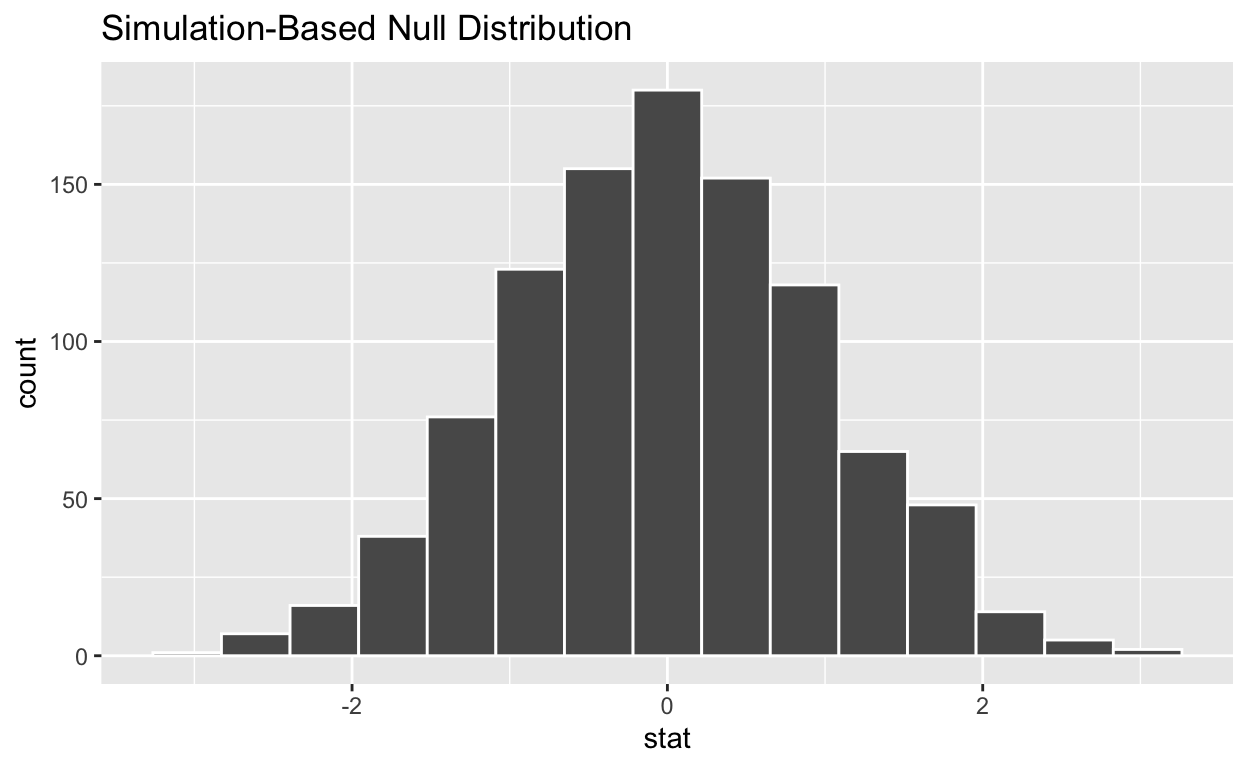calculate the statistic from your observed data

• Assign the output observed_t_statistic

• Display observed_t_statistic

`````` observed_t_statistic  <- hr  %>%
specify(response = hours)  %>%
hypothesize(null = "point", mu = 48)  %>%
calculate(stat = "t")

observed_t_statistic
``````
``````# A tibble: 1 x 1
stat
<dbl>
1  2.37``````

get_p_value from the simulated null distribution and the observed statistic

``````null_t_distribution  %>%
get_p_value (obs_stat = observed_t_statistic , direction = "two-sided")
``````
``````# A tibble: 1 x 1
p_value
<dbl>
1   0.018``````

shade_p_value on the simulated null distribution

``````null_t_distribution  %>%
visualize() +
shade_p_value(obs_stat = observed_t_statistic, direction = "two-sided")
``````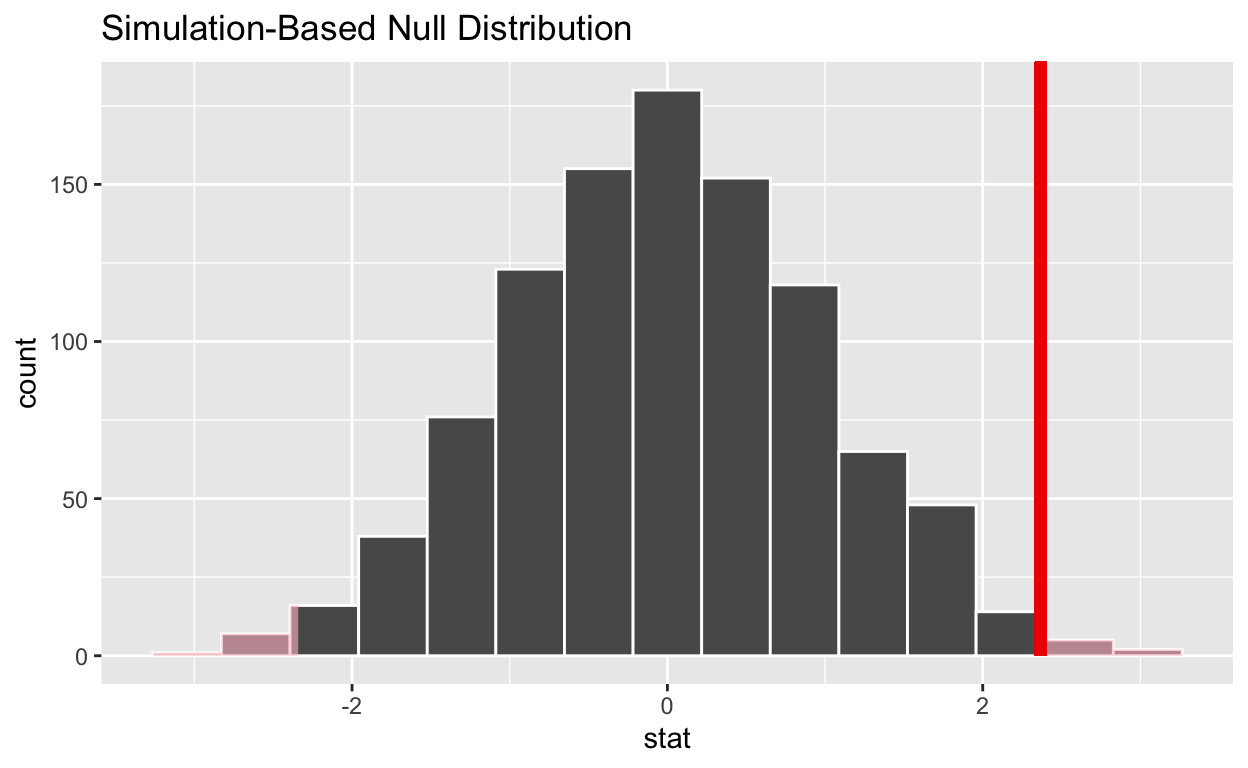If the p-value < 0.05? no (yes/no)

Does your analysis support the null hypothesis that the true mean number of hours worked was 48? no (yes/no)

# Question: 2 sample t-test

SEE QUIZ is the name of your data subset

• Read it into and assign to hr_2

• Note: col_types = “fddfff” defines the column types factor-double-double-factor-factor-factor
``````hr_2 <- read_csv("https://estanny.com/static/week13/data/hr_2_tidy.csv",
col_types = "fddfff")
``````

# Q: Is the average number of hours worked the same for both genders in hr_2?

use skim to summarize the data in hr_2 by gender

``````hr_2 %>%
group_by(gender)  %>%
skim()
``````
 Name Piped data Number of rows 500 Number of columns 6 _______________________ Column type frequency: factor 3 numeric 2 ________________________ Group variables gender

Variable type: factor

skim_variable gender n_missing complete_rate ordered n_unique top_counts
evaluation male 0 1 FALSE 4 bad: 79, fai: 68, goo: 61, ver: 48
evaluation female 0 1 FALSE 4 bad: 75, fai: 74, ver: 48, goo: 47
salary male 0 1 FALSE 6 lev: 49, lev: 48, lev: 48, lev: 44
salary female 0 1 FALSE 6 lev: 47, lev: 46, lev: 41, lev: 39
status male 0 1 FALSE 3 fir: 93, pro: 90, ok: 73
status female 0 1 FALSE 3 fir: 101, pro: 89, ok: 54

Variable type: numeric

skim_variable gender n_missing complete_rate mean sd p0 p25 p50 p75 p100 hist
age male 0 1 38.63 11.57 20.3 28.50 37.85 49.52 59.6 ▇▇▆▆▆
age female 0 1 41.14 11.43 20.3 31.30 41.60 50.90 59.9 ▆▅▇▇▇
hours male 0 1 49.30 13.24 35.0 37.35 46.00 59.23 79.9 ▇▃▂▂▂
hours female 0 1 49.49 13.08 35.0 37.68 45.05 58.73 78.4 ▇▃▃▂▂
• Females worked an average of 49.5 hours per week

• Males worked an average of 49.3 hours per week

Use geom_boxplot to plot distributions of hours worked by gender

``````hr_2 %>%
ggplot(aes(x = gender, y = hours)) +
geom_boxplot()
``````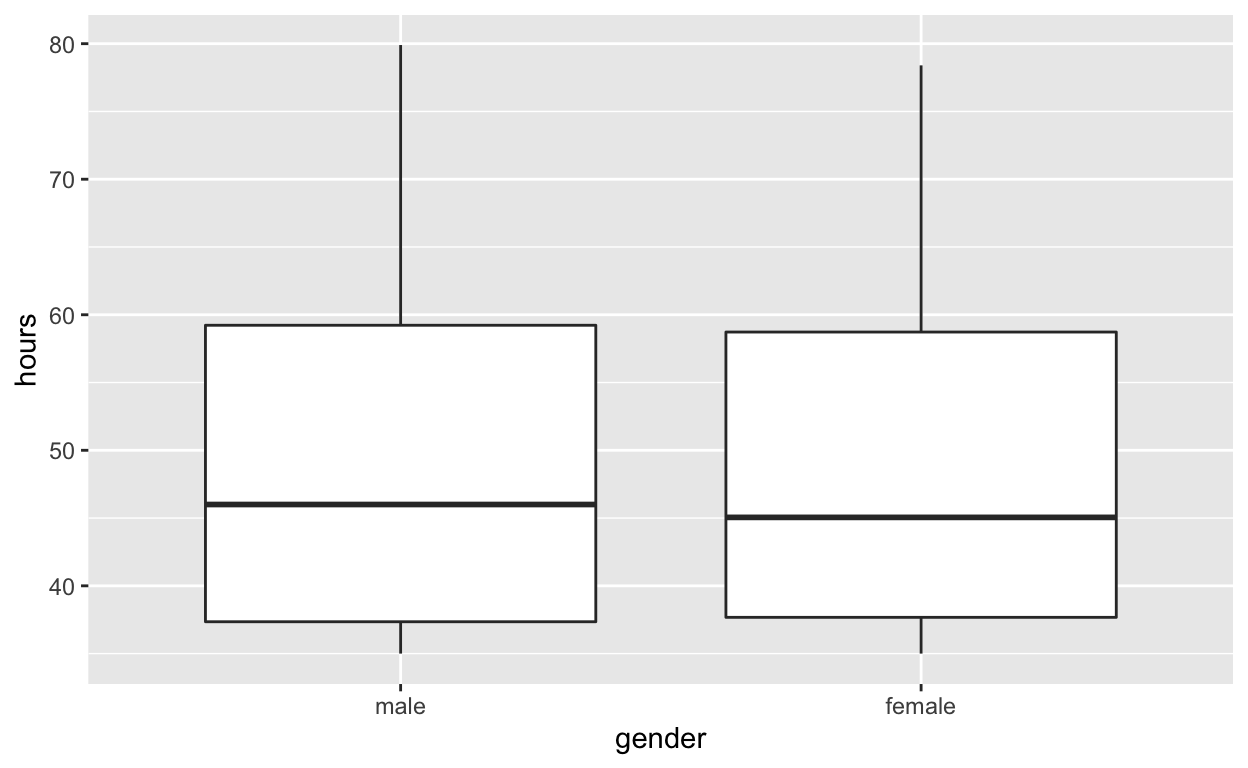specify the variables of interest are hours and gender

``````hr_2 %>%
specify(response = hours, explanatory = gender)
``````
``````Response: hours (numeric)
Explanatory: gender (factor)
# A tibble: 500 x 2
hours gender
<dbl> <fct>
1  78.1 male
2  35.1 female
3  36.9 female
4  38.5 male
5  36.1 male
6  78.1 female
7  76   female
8  35.6 female
9  35.6 male
10  56.8 male
# … with 490 more rows``````

hypothesize that the number of hours worked and gender are independent

``````hr_2  %>%
specify(response = hours, explanatory = gender)  %>%
hypothesize(null = "independence")
``````
``````Response: hours (numeric)
Explanatory: gender (factor)
Null Hypothesis: independence
# A tibble: 500 x 2
hours gender
<dbl> <fct>
1  78.1 male
2  35.1 female
3  36.9 female
4  38.5 male
5  36.1 male
6  78.1 female
7  76   female
8  35.6 female
9  35.6 male
10  56.8 male
# … with 490 more rows``````

generate 1000 replicates representing the null hypothesis

``````hr_2 %>%
specify(response = hours, explanatory = gender)  %>%
hypothesize(null = "independence")  %>%
generate(reps = 1000, type = "permute")
``````
``````Response: hours (numeric)
Explanatory: gender (factor)
Null Hypothesis: independence
# A tibble: 500,000 x 3
# Groups:   replicate [1,000]
hours gender replicate
<dbl> <fct>      <int>
1  60.1 male           1
2  50.2 female         1
3  51.9 female         1
4  42.9 male           1
5  58.3 male           1
6  53.7 female         1
7  48.5 female         1
8  41.9 female         1
9  65.8 male           1
10  45.1 male           1
# … with 499,990 more rows``````

The output has 500,000 rows

calculate the distribution of statistics from the generated data

• Assign the output null_distribution_2_sample_permute

• Display null_distribution_2_sample_permute

``````null_distribution_2_sample_permute  <- hr_2 %>%
specify(response = hours, explanatory = gender)  %>%
hypothesize(null = "independence")  %>%
generate(reps = 1000, type = "permute")  %>%
calculate(stat = "F", order = c("female", "male"))

null_distribution_2_sample_permute
``````
``````# A tibble: 1,000 x 2
replicate    stat
*     <int>   <dbl>
1         1 0.858
2         2 0.0917
3         3 0.0217
4         4 0.00763
5         5 0.0169
6         6 0.247
7         7 2.43
8         8 0.918
9         9 1.91
10        10 0.413
# … with 990 more rows``````

null_t_distribution has 2000 t-stats

visualize the simulated null distribution

``````visualize(null_distribution_2_sample_permute)
``````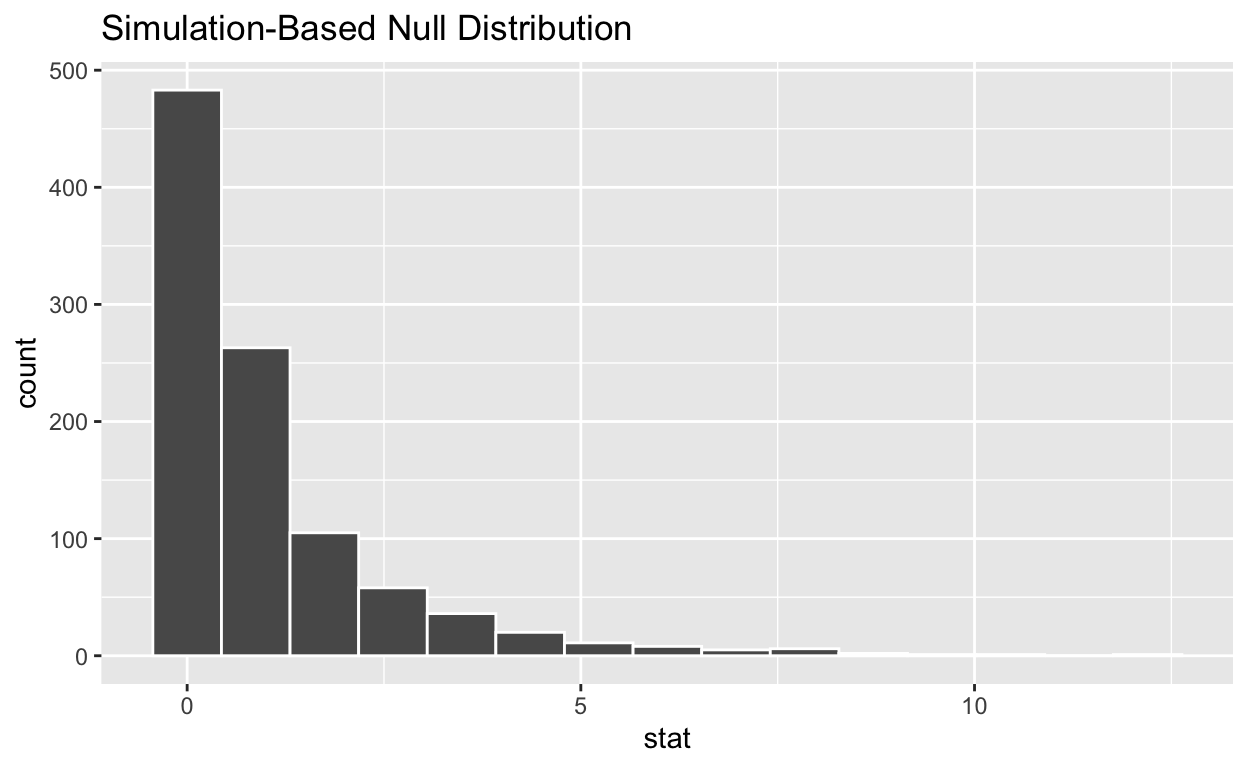calculate the statistic from your observed data

• Assign the output observed_t_2_sample_stat

• Display observed_t_2_sample_stat

``````observed_t_2_sample_stat  <- hr_2 %>%
specify(response = hours, explanatory = gender)  %>%
calculate(stat = "t", order = c("female", "male"))

observed_t_2_sample_stat
``````
``````# A tibble: 1 x 1
stat
<dbl>
1 0.160``````

get_p_value from the simulated null distribution and the observed statistic

``````null_t_distribution  %>%
get_p_value(obs_stat = observed_t_2_sample_stat , direction = "two-sided")
``````
``````# A tibble: 1 x 1
p_value
<dbl>
1    0.84``````

shade_p_value on the simulated null distribution

``````null_t_distribution  %>%
visualize() +
shade_p_value(obs_stat = observed_t_2_sample_stat, direction = "two-sided")
``````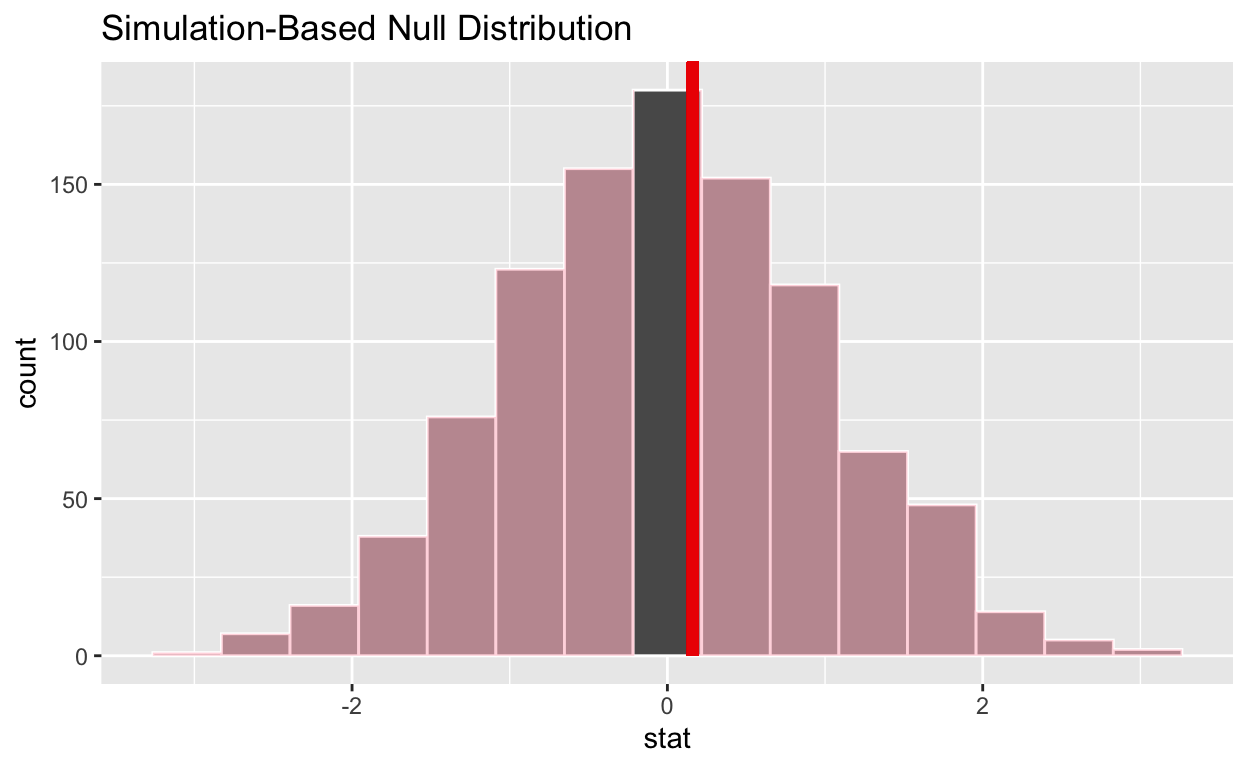If the p-value < 0.05? no (yes/no)

Does your analysis support the null hypothesis that the true mean number of hours worked by female and male employees was the same? yes (yes/no)

# Question: ANOVA

SEE QUIZ is the name of your data subset

Read it into and assign to hr_anova

Note: col_types = “fddfff” defines the column types factor-double-double-factor-factor-factor

``````hr_anova <- read_csv("https://estanny.com/static/week13/data/hr_3_tidy.csv",
col_types = "fddfff")
``````

# Q: Is the average number of hours worked the same for all three status (fired, ok and promoted) ?

use skim to summarize the data in hr_anova by status

``````hr_anova %>%
group_by(status)  %>%
skim()
``````
 Name Piped data Number of rows 500 Number of columns 6 _______________________ Column type frequency: factor 3 numeric 2 ________________________ Group variables status

Variable type: factor

skim_variable status n_missing complete_rate ordered n_unique top_counts
gender promoted 0 1 FALSE 2 fem: 91, mal: 81
gender fired 0 1 FALSE 2 mal: 98, fem: 98
gender ok 0 1 FALSE 2 mal: 68, fem: 64
evaluation promoted 0 1 FALSE 4 goo: 79, ver: 52, fai: 21, bad: 20
evaluation fired 0 1 FALSE 4 bad: 77, fai: 64, ver: 30, goo: 25
evaluation ok 0 1 FALSE 4 fai: 53, bad: 51, goo: 18, ver: 10
salary promoted 0 1 FALSE 6 lev: 42, lev: 37, lev: 36, lev: 28
salary fired 0 1 FALSE 6 lev: 59, lev: 40, lev: 39, lev: 25
salary ok 0 1 FALSE 6 lev: 33, lev: 29, lev: 28, lev: 23

Variable type: numeric

skim_variable status n_missing complete_rate mean sd p0 p25 p50 p75 p100 hist
age promoted 0 1 40.22 11.11 20.1 31.67 41.00 48.82 59.7 ▆▇▇▇▇
age fired 0 1 38.95 11.23 20.0 29.82 38.80 48.75 59.9 ▇▆▇▇▅
age ok 0 1 39.03 11.77 20.0 28.28 38.75 49.92 59.7 ▇▇▆▇▆
hours promoted 0 1 59.29 12.53 35.0 49.90 58.65 70.35 79.9 ▅▆▇▆▇
hours fired 0 1 42.37 9.15 35.0 36.20 39.20 43.80 79.6 ▇▁▁▁▁
hours ok 0 1 47.99 11.55 35.0 37.45 45.75 55.23 75.7 ▇▃▃▂▂

Employees that were fired worked an average of 42.4 hours per week

Employees that were ok worked an average of 48.0 hours per week

Employees that were promoted worked an average of 59.3 hours per week

Use geom_boxplot to plot distributions of hours worked by status

``````hr_anova %>%
ggplot(aes(x = status, y = hours)) +
geom_boxplot()
``````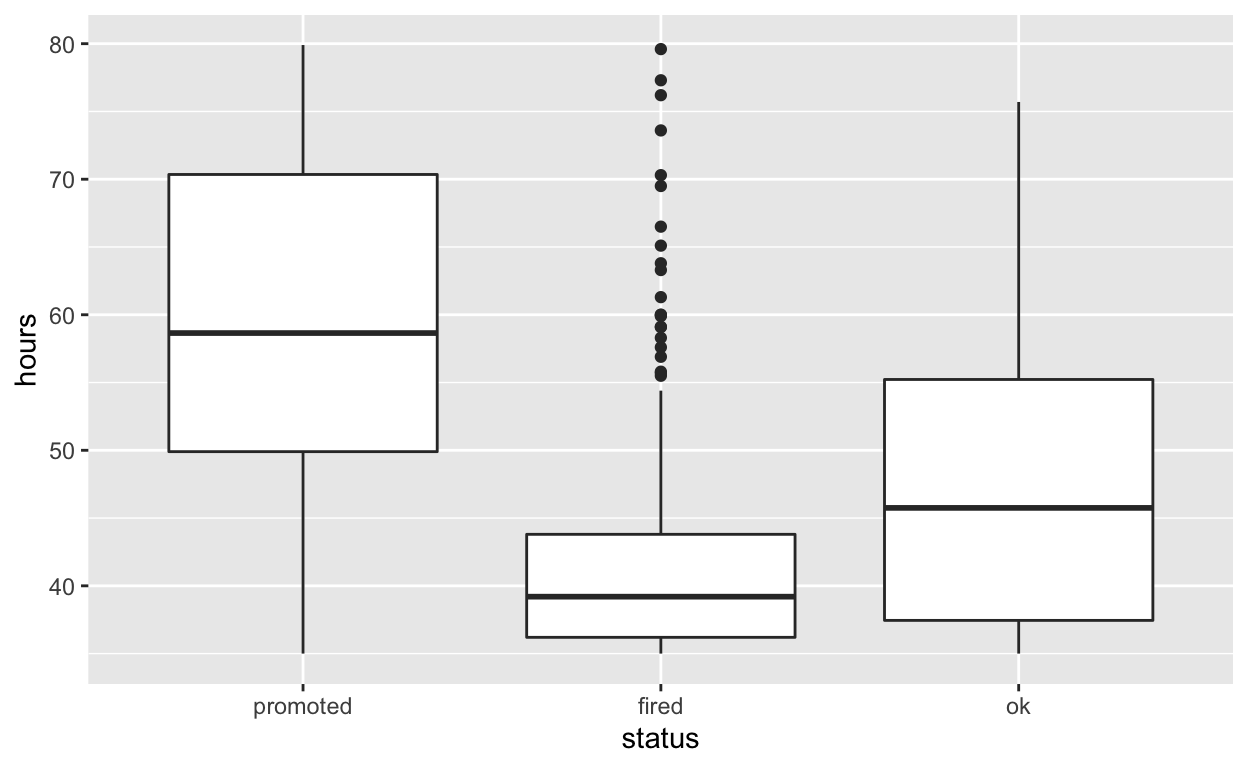specify the variables of interest are hours and status

``````hr_anova %>%
specify(response = hours, explanatory = status)
``````
``````Response: hours (numeric)
Explanatory: status (factor)
# A tibble: 500 x 2
hours status
<dbl> <fct>
1  49.6 promoted
2  39.2 fired
3  63.2 promoted
4  42.2 promoted
5  54.7 promoted
6  54.3 fired
7  37.3 fired
8  45.6 promoted
9  35.1 fired
10  53   promoted
# … with 490 more rows``````

hypothesize that the number of hours worked and status are independent

``````hr_anova  %>%
specify(response = hours, explanatory = status)  %>%
hypothesize(null = "independence")
``````
``````Response: hours (numeric)
Explanatory: status (factor)
Null Hypothesis: independence
# A tibble: 500 x 2
hours status
<dbl> <fct>
1  49.6 promoted
2  39.2 fired
3  63.2 promoted
4  42.2 promoted
5  54.7 promoted
6  54.3 fired
7  37.3 fired
8  45.6 promoted
9  35.1 fired
10  53   promoted
# … with 490 more rows``````

generate 1000 replicates representing the null hypothesis

``````hr_anova %>%
specify(response = hours, explanatory = status)  %>%
hypothesize(null = "independence")  %>%
generate(reps = 1000, type = "permute")
``````
``````Response: hours (numeric)
Explanatory: status (factor)
Null Hypothesis: independence
# A tibble: 500,000 x 3
# Groups:   replicate [1,000]
hours status   replicate
<dbl> <fct>        <int>
1  53.1 promoted         1
2  39.2 fired            1
3  70.3 promoted         1
4  35   promoted         1
5  67.6 promoted         1
6  71.4 fired            1
7  43.4 fired            1
8  39.4 promoted         1
9  37.6 fired            1
10  37.5 promoted         1
# … with 499,990 more rows``````

The output has 500,000 rows

calculate the distribution of statistics from the generated data

• Assign the output null_distribution_anova

• Display null_distribution_anova

``````null_distribution_anova  <- hr_anova %>%
specify(response = hours, explanatory = gender)  %>%
hypothesize(null = "independence")  %>%
generate(reps = 1000, type = "permute")  %>%
calculate(stat = "F")

null_distribution_anova
``````
``````# A tibble: 1,000 x 2
replicate    stat
*     <int>   <dbl>
1         1 0.737
2         2 4.92
3         3 0.656
4         4 0.190
5         5 0.399
6         6 0.00942
7         7 4.44
8         8 0.127
9         9 0.945
10        10 1.38
# … with 990 more rows``````

null_distribution_anova has 1000 F-stats

visualize the simulated null distribution

``````visualize(null_distribution_anova)
``````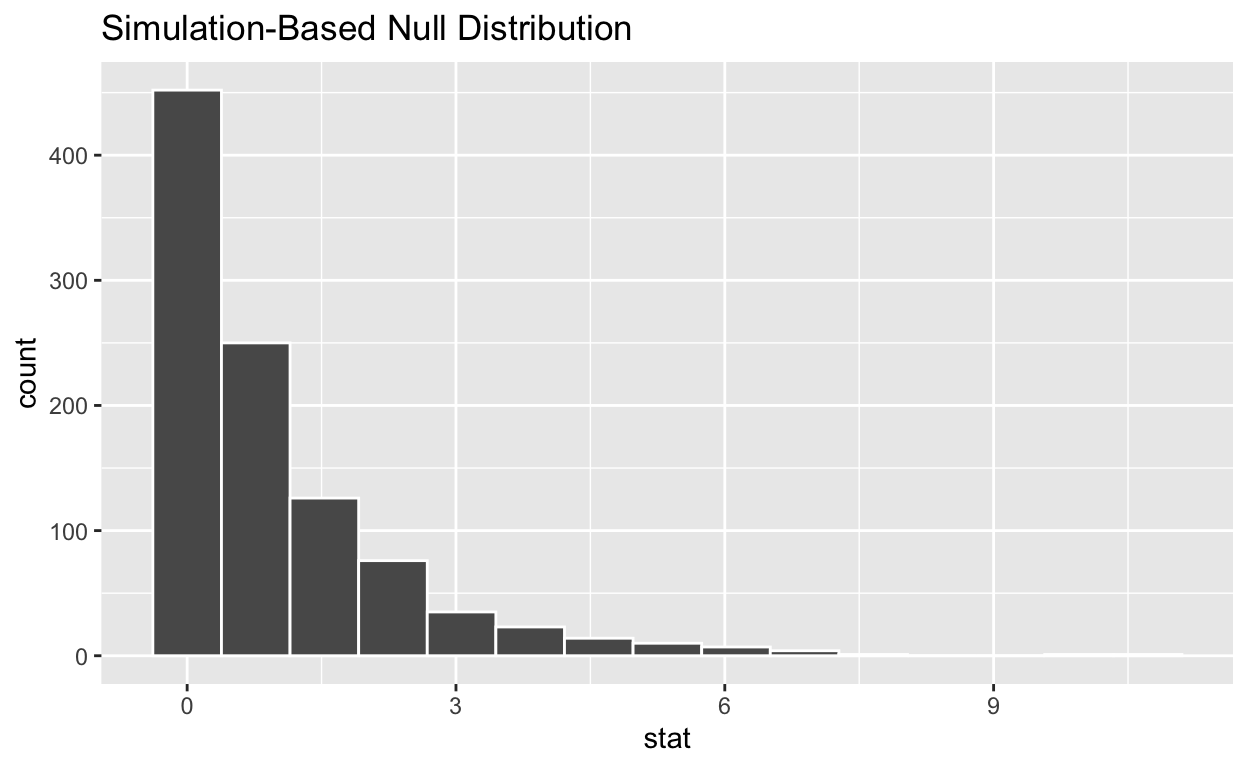calculate the statistic from your observed data

Assign the output observed_f_sample_stat

Display observed_f_sample_stat

``````observed_f_sample_stat  <- hr_anova %>%
specify(response = hours, explanatory = status)  %>%
calculate(stat = "F")

observed_f_sample_stat
``````
``````# A tibble: 1 x 1
stat
<dbl>
1  110.``````

get_p_value from the simulated null distribution and the observed statistic

``````null_distribution_anova  %>%
get_p_value(obs_stat = null_distribution_anova , direction = "greater")
``````
``````# A tibble: 1 x 1
p_value
<dbl>
1   0.328``````

shade_p_value on the simulated null distribution

``````null_t_distribution  %>%
visualize() +
shade_p_value(obs_stat = null_distribution_anova, direction = "greater")
``````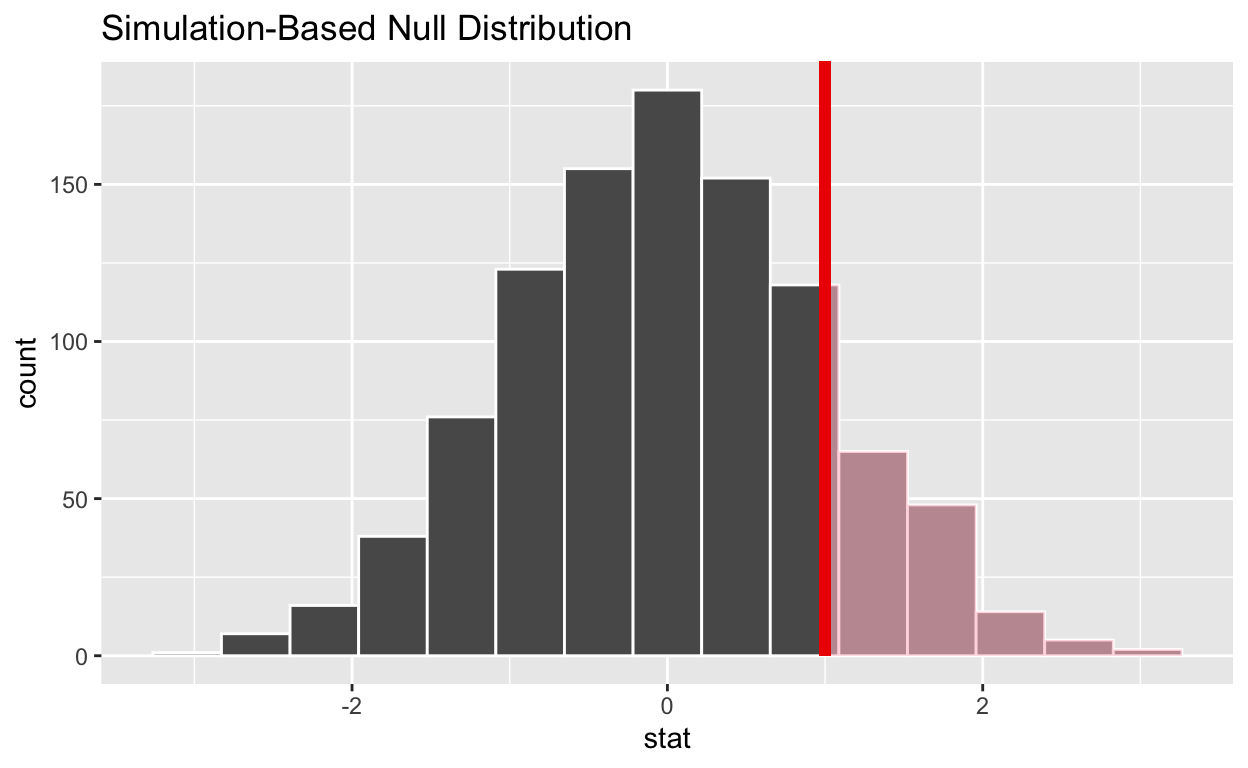If the p-value < 0.05? yes (yes/no)

Does your analysis support the null hypothesis that the true means of the number of hours worked for those that were “fired”, “ok” and “promoted” were the same? no (yes/no)